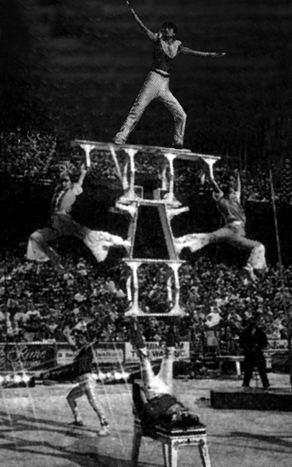Select Chapter Topics:A structural steel rod has a radius of 10 mm and a length of 1.0 m. A 100 kN force stretches it along its length. The stress produced and the elongation in the rod, respectively, are?

(Young’s modulus of structural steel is )

1.

2.

3.

4.Subtopic:  Hooke's Law |
67%
From NCERT
To view explanation, please take trial in the course.
NEET 2023 - Target Batch - Aryan Raj Singh
Hints
To view explanation, please take trial in the course.
NEET 2023 - Target Batch - Aryan Raj Singh

A copper wire of length 2.2 m and a steel wire of length 1.6 m, both of diameter 3.0 mm, are connected end to end. When stretched by a load, the net elongation is found to be 0.70 mm. The load applied is:

YC  = 1.1 × 1011 N m2 and YS = 2.0 × 1011 N m-2
1.

2.

3.

4.Subtopic:  Hooke's Law |
From NCERT
To view explanation, please take trial in the course.
NEET 2023 - Target Batch - Aryan Raj Singh
Hints
To view explanation, please take trial in the course.
NEET 2023 - Target Batch - Aryan Raj Singh

In a human pyramid in a circus, the entire weight of the balanced group is supported by the legs of a performer who is lying on his back (as shown in the figure below). The combined mass of all the persons performing the act, and the tables, plaques, etc. involved is 280 kg. The mass of the performer lying on his back at the bottom of the pyramid is 60 kg. Each thighbone (femur) of this performer has a length of 50 cm and an effective radius of 2.0 cm. The amount by which each thighbone gets compressed under the extra load is:

(The Young’s modulus for bone is given by, Y = )1.

2.

3.

4.Subtopic:  Stress - Strain |
From NCERT
To view explanation, please take trial in the course.
NEET 2023 - Target Batch - Aryan Raj Singh
Hints
To view explanation, please take trial in the course.
NEET 2023 - Target Batch - Aryan Raj Singh

A square lead slab of side 50 cm and thickness 10 cm is subject to a shearing force (on its narrow face) of $9.0×{10}^{4}$ $\mathrm{N}$. The lower edge is riveted to the floor as shown in the figure below. How much will the upper edge be displaced?

$\left(\mathrm{Shear}\right)$ $\mathrm{modulus}$ $\mathrm{of}$ $\mathrm{lead}$ $=$ $5.6×{10}^{9}$ $\mathrm{N}$ ${\mathrm{m}}^{-2}$1.   0.16 mm

2.   1.8 mm

3.   18 mm

4.   16 mmSubtopic:  Shear and bulk modulus |
58%
From NCERT
To view explanation, please take trial in the course.
NEET 2023 - Target Batch - Aryan Raj Singh
Hints
To view explanation, please take trial in the course.
NEET 2023 - Target Batch - Aryan Raj Singh

The average depth of the Indian Ocean is about $$3000$$ m. The fractional compression, $$\frac{\Delta V}{V},$$ of water at the bottom of the ocean is?
(Given that the bulk modulus of water is $$2.2\times10^{9}$$ Nm-2 and $$g=10$$ ms-2)
1. $$1.36\times10^{-3}$$
2. $$2.36\times10^{-3}$$
3. $$1.36\times10^{-2}$$
4. $$2.36\times10^{-2}$$Subtopic:  Shear and bulk modulus |
71%
From NCERT
To view explanation, please take trial in the course.
NEET 2023 - Target Batch - Aryan Raj Singh
Hints